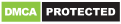# RxJS Observable Pipe, Subscribe, Map and Filter Examples with Angular 9/8

In this tutorial we'll learn by example to use the RxJS' `pipe()` function, the `map()` and `filter()` operators in Angular 9. And how to use the `subscribe()` method to subscribe to Observables.

RxJS' `pipe()` is both a standalone function and a method on the Observable interface that can be used to combine multiple RxJS operators to compose asynchronous operations.

The `pipe()` function takes one or more operators and returns an RxJS Observable.

`pipe()` takes a bunch of RxJS operators as arguments such as `filter` and `map`separated by comma and run them in the sequence they are added and finally returns an `RxJS Observable`. To get the result we need to `subscribe()` to the returned Observable.

Note: `pipe()` is a function/method that is used to chain multiple RxJS operators while `map()` and `filter()` are operators that operate and transform the values of an Observable (sequence of values). They are similar to the `map()` and `filter()` methods of JavaScript arrays.

Let's see this by example.

First, let's add the following imports:

``````import { of, Observable } from 'rxjs';
import { filter, map } from 'rxjs/operators';
``````

Next, we need to create an Observable using the `of()` function from a sequence of 1 to 10 numbers and use the pipe() method to apply the `filter()` operator on the sequence:

``````const ob\$: Observable<number> = of(1, 2, 3, 4, 5, 6, 7, 8, 9, 10).pipe(filter( v => v % 2 === 0));
``````

The `filter()` operator filters the seqeunce and returns a new sequence of the values that verify the `v => v % 2 === 0` predicate i.e only even numbers.

Finally, let's run this by subscribing to the returned Observable:

``````ob\$.subscribe(
next => console.log('next:', next),
err => console.log('error:', err),
() => console.log('Completed'),
);
``````

This is the output: ``` next: 2 next: 4 next: 6 next: 8 next: 10 Completed ```

Next, let's apply the `map()` operator to the sequence as follows:

``````const ob\$: Observable<number> = of(1, 2, 3, 4, 5, 6, 7, 8, 9, 10).pipe(filter( v => v % 2 === 0), map( v => v * 10));
``````

We apply both the `filter()` and `map()` operators, `filter()` will be executed first then `map()`. This will produce the following output:

``````next: 20
next: 40
next: 60
next: 80
next: 100
Completed
``````

`map()` transforms each value of the source Observable using the passed formula. In our case, `v => v * 10` i.e it multiplies each value by ten.

## Angular 9 Example with RxJS' `pipe()`, `map()` and `filter()`

Let's now see how to use `pipe()`, `map()` and `filter()` in real Angular 9 use case.

Let's start by genrating a new Angular service using the following command:

``````\$ ng g service country
``````

Next, open the `src/app/country.service.ts` file and add the following imports:

``````
``````